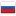Benchmarks of different segment tree implementations
Difference between en1 and en2, changed 8 character(s)
Sometimes I wonder, what implementation of a segment tree to use. Usually, I handwave that some will suit, and that works in most of the cases.↵

But today I decided to back up the choice with some data and I ran benchmarks against 4 implementations:↵
<ul>↵
<li>↵
Simple recursive divide-and-conquer implementation↵

<spoiler summary="Code">↵
~~~↵
struct SimpleRecursiveSegmentTree {↵
unsigned size;↵

private:↵
std::vector<long long> t;↵

void _build(const std::vector<int> &v, unsigned p, unsigned l, unsigned r) {↵
if (r == l + 1) {↵
t[p] = v[l];↵
return;↵
}↵
unsigned m = (l + r) / 2;↵
_build(v, 2 * p + 1, l, m);↵
_build(v, 2 * p + 2, m, r);↵
t[p] = t[2 * p + 1] + t[2 * p + 2];↵
}↵

long long _get(unsigned p, unsigned l, unsigned r, unsigned a,↵
unsigned b) const {↵
if (b <= l || r <= a) {↵
return 0LL;↵
}↵
if (a <= l && r <= b) {↵
return t[p];↵
}↵
unsigned m = (l + r) / 2;↵
return _get(2 * p + 1, l, m, a, b) + _get(2 * p + 2, m, r, a, b);↵
}↵

void _add(unsigned p, unsigned l, unsigned r, unsigned i, int x) {↵
if (i < l || r <= i) {↵
return;↵
}↵
if (r == l + 1) {↵
t[p] += x;↵
return;↵
}↵
unsigned m = (l + r) / 2;↵
_add(2 * p + 1, l, m, i, x);↵
_add(2 * p + 2, m, r, i, x);↵
t[p] = t[2 * p + 1] + t[2 * p + 2];↵
}↵

public:↵
SimpleRecursiveSegmentTree(unsigned _size) noexcept : size(_size) {↵
t.resize(4 * size);↵
}↵

SimpleRecursiveSegmentTree(const std::vector<int> &v) noexcept↵
: size(v.size()) {↵
t.resize(4 * size);↵
_build(v, 0, 0, size);↵
}↵

void add(unsigned i, int x) { _add(0, 0, size, i, x); }↵

long long get(unsigned l, unsigned r) const {↵
return _get(0, 0, size, l, r);↵
}↵
};↵
~~~↵
</spoiler>↵

</li>↵
<li>↵
Optimized recursive divide-and-conquer, which does not descend into apriori useless branches↵

<spoiler summary="Code">↵
~~~↵
struct OptimizedRecursiveSegmentTree {↵
unsigned size;↵

private:↵
std::vector<long long> t;↵

void _build(const std::vector<int> &v, unsigned p, unsigned l, unsigned r) {↵
if (r == l + 1) {↵
t[p] = v[l];↵
return;↵
}↵
unsigned m = (l + r) / 2;↵
_build(v, 2 * p + 1, l, m);↵
_build(v, 2 * p + 2, m, r);↵
t[p] = t[2 * p + 1] + t[2 * p + 2];↵
}↵

long long _get(unsigned p, unsigned l, unsigned r, unsigned a,↵
unsigned b) const {↵
if (a <= l && r <= b) {↵
return t[p];↵
}↵
unsigned m = (l + r) / 2;↵
long long res = 0;↵
if (a < m) {↵
res += _get(2 * p + 1, l, m, a, b);↵
}↵
if (b > m) {↵
res += _get(2 * p + 2, m, r, a, b);↵
}↵
return res;↵
}↵

void _add(unsigned p, unsigned l, unsigned r, unsigned i, int x) {↵
if (r == l + 1) {↵
t[p] += x;↵
return;↵
}↵
unsigned m = (l + r) / 2;↵
if (i < m) {↵
_add(2 * p + 1, l, m, i, x);↵
} else {↵
_add(2 * p + 2, m, r, i, x);↵
}↵
t[p] = t[2 * p + 1] + t[2 * p + 2];↵
}↵

public:↵
OptimizedRecursiveSegmentTree(unsigned _size) noexcept : size(_size) {↵
t.resize(4 * size);↵
}↵

OptimizedRecursiveSegmentTree(const std::vector<int> &v) noexcept↵
: size(v.size()) {↵
t.resize(4 * size);↵
_build(v, 0, 0, size);↵
}↵

void add(unsigned i, int x) { _add(0, 0, size, i, x); }↵

long long get(unsigned l, unsigned r) const {↵
return _get(0, 0, size, l, r);↵
}↵
};↵
~~~↵
</spoiler>↵

</li>↵
<li>↵
Non-recursive implementation (credits: https://codeforces.com/blog/entry/18051)↵

<spoiler summary="Code">↵
~~~↵
struct NonRecursiveSegmentTree {↵
unsigned size;↵

private:↵
std::vector<long long> t;↵

void _build(const std::vector<int> &v) {↵
std::copy(v.begin(), v.end(), t.begin() + size);↵
for (int i = size - 1; i > 0; --i) {↵
t[i] = t[i * 2] + t[i * 2 ^ 1];↵
}↵
}↵

public:↵
NonRecursiveSegmentTree(unsigned _size) noexcept : size(_size) {↵
t.resize(2 * size);↵
}↵

NonRecursiveSegmentTree(const std::vector<int> &v) noexcept↵
: size(v.size()) {↵
t.resize(2 * size);↵
_build(v);↵
}↵

void add(unsigned i, int x) {↵
i += size;↵
for (t[i] += x; i > 1; i /= 2) {↵
t[i / 2] = t[i] + t[i ^ 1];↵
}↵
}↵

long long get(unsigned l, unsigned r) const {↵
long long res = 0;↵
for (l += size, r += size; l < r; l /= 2, r /= 2) {↵
if (l & 1) {↵
res += t[l++];↵
}↵
if (r & 1) {↵
res += t[--r];↵
}↵
}↵
return res;↵
}↵
};↵
~~~↵
</spoiler>↵

</li>↵
<li>↵
Fenwick Tree↵

<spoiler summary="Code">↵
~~~↵
struct FenwickTree {↵
unsigned size;↵

private:↵
std::vector<long long> t;↵

long long get_prefix(int i) const {↵
long long res = 0;↵
while (i >= 0) {↵
res += t[i];↵
i = (i & (i + 1)) - 1;↵
}↵
return res;↵
}↵

public:↵
void add(unsigned i, int x) {↵
while (i < size) {↵
t[i] += x;↵
i = i | (i + 1);↵
}↵
}↵

FenwickTree(unsigned _size) : size(_size) { t.resize(size); }↵

FenwickTree(const std::vector<int> &v) : size(v.size()) {↵
t.resize(size);↵
for (unsigned i = 0; i < size; ++i) {↵
}↵
}↵

long long get(unsigned l, unsigned r) {↵
return get_prefix((int)r - 1) - get_prefix((int)l - 1);↵
}↵
};↵
~~~↵
</spoiler>↵

</li>↵
</ul>↵

All implementations are able to:↵
<ul>↵
<li>↵
get(l, r): get sum on a segment (half-interval) $[l; r)$↵
</li>↵
<li>↵
update(i, x): add $x$ to the element by index $i$↵
</li>↵
</ul>↵

Here are the results:↵

Note: I decided not to apply any addition-specific optimizations so that with minor modifications the data structures could be used for any supported operations.↵

I generated queries the following way:↵

- Update: generate random index (rnd() % size) and number↵
- Query: at first, generate random length (rnd() % size + 1), then generate a left border for that length↵

[Benchmarking source code](https://gist.github.com/pavel-the-best/35e1bf7759152c442f3c83d721947e97#file-bench-cpp). Note that ideally you should disable frequency scaling and close any applications that might disrupt the benchmark (basically, the more you close &mdash; the better).↵

[Plot-generating Python script](https://gist.github.com/pavel-the-best/8636aeb68db2119b00fce679d361df6f#file-generate_plot-py)↵

[My benchmarking data](https://gist.github.com/pavel-the-best/f33879ea075b5e9b3ea16e4d85d807dd) in case you want to do some more plotting/comparing.↵

I compiled the benchmark with #pragma GCC optimize("O3") on GNU GCC 11.3.0, and ran it with the CPU fixed on 2.4 GHz without the desktop environment open.↵

This is probably my first useful contribution so any suggestions/improvements are welcome.↵

#### History

RevisionsRev. Lang. By When Δ Comment
en4pavelthebest 2022-07-01 18:47:14 227 Benchmark data updated: benchmark process was pinned on a single CPU core
ru4pavelthebest 2022-07-01 18:41:47 205 Результаты обновлены: бенчмарк был запущен с процессом "прибитым" к одному ядру процессора
en3pavelthebest 2022-07-01 00:42:53 7 Tiny change: '>\n<li>\nupdate(i, x): a' -> '>\n<li>\nadd(i, x): a'
ru3pavelthebest 2022-07-01 00:42:11 7 Мелкая правка: '>\n<li>\nupdate(i, x): п' -> '>\n<li>\nadd(i, x): п'
ru2pavelthebest 2022-06-30 23:40:36 4 (опубликовано)
en2pavelthebest 2022-06-30 23:40:01 8 (published)
ru1pavelthebest 2022-06-30 23:39:25 7722 Первая редакция перевода на Русский (сохранено в черновиках)
en1pavelthebest 2022-06-30 23:27:37 7598 Initial revision (saved to drafts)